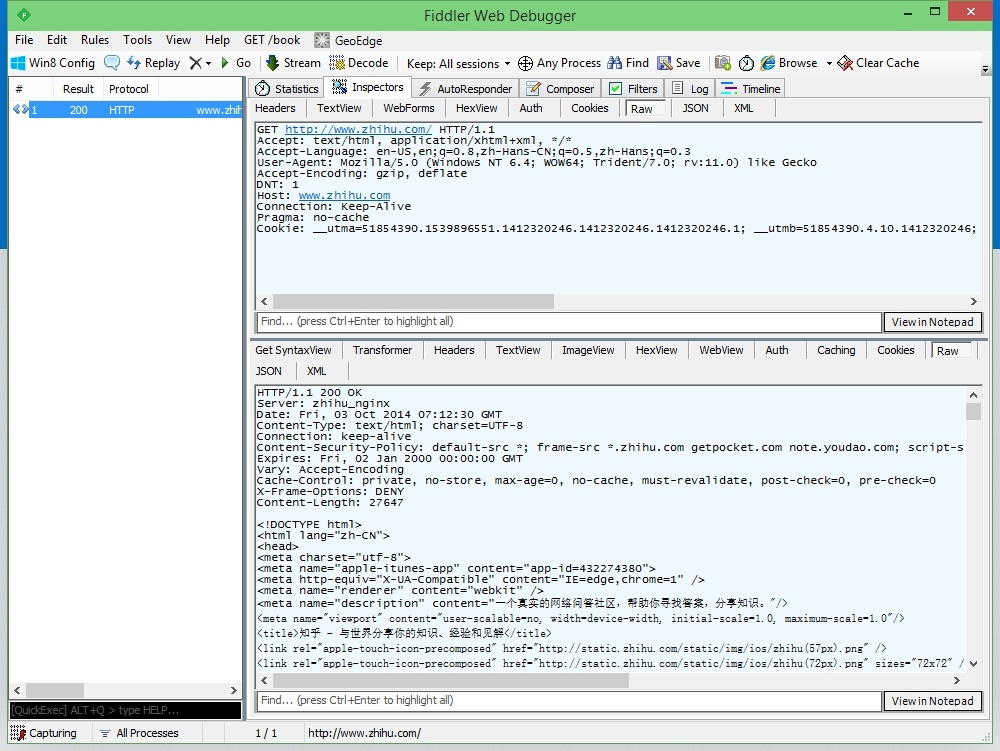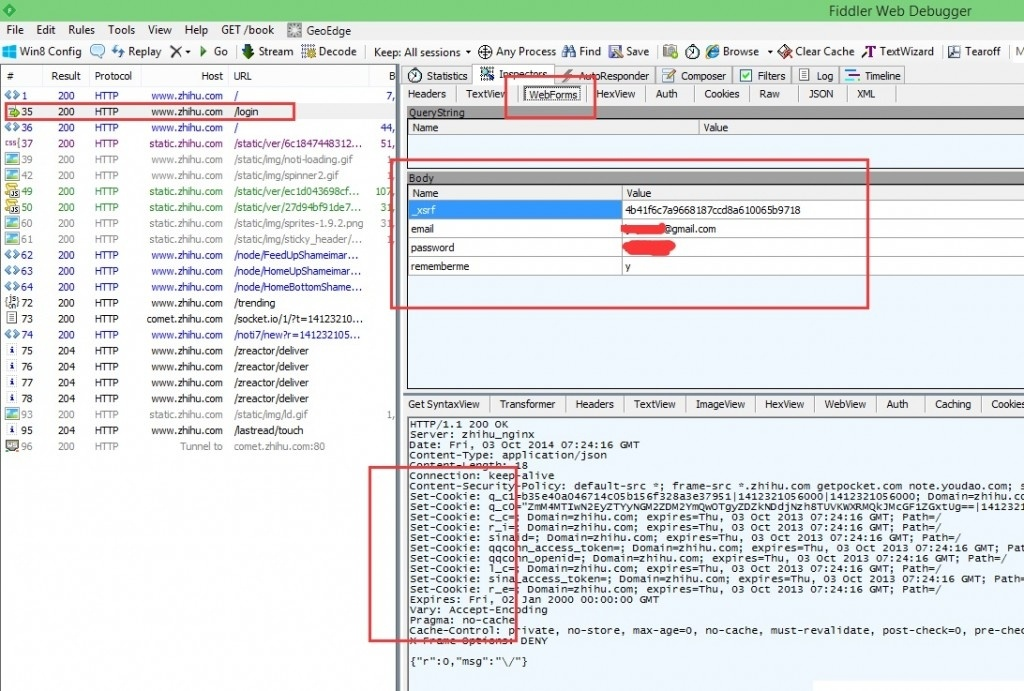# 零基础自学用Python 3开发网络爬虫(四): 登录

## 第一步: 使用 Fiddler 观察浏览器行为``````POST http://www.zhihu.com/login HTTP/1.1

Content-Type: application/x-www-form-urlencoded; charset=UTF-8
Accept: \*/\*
X-Requested-With: XMLHttpRequest
Referer: http://www.zhihu.com/#signin
Accept-Language: en-US,en;q=0.8,zh-Hans-CN;q=0.5,zh-Hans;q=0.3
Accept-Encoding: gzip, deflate
User-Agent: Mozilla/5.0 (Windows NT 6.4; WOW64; Trident/7.0; rv:11.0) like Gecko
Content-Length: 97
DNT: 1
Host: www.zhihu.com
Connection: Keep-Alive
Pragma: no-cache

Cookie: \_\_utma=51854390.1539896551.1412320246.1412320246.1412320246.1; \_\_utmb=51854390.6.10.1412320246; \_\_utmc=51854390; \_\_utmz=51854390.1412320246.1.1.utmcsr=(direct)|utmccn=(direct)|utmcmd=(none); _\_utmv=51854390.000--|3=entry\_date=20141003=1

``````## 第二步: 解压缩

``````import gzip
def ungzip(data):
try:        # 尝试解压
print('正在解压.....')
data = gzip.decompress(data)
print('解压完毕!')
except:
print('未经压缩, 无需解压')
return data
``````

## 第二步: 使用正则表达式获取沙漠之舟

_xsrf 这个键的值在茫茫无际的互联网沙漠之中指引我们用正确的姿势来登录知乎, 所以 _xsrf 可谓沙漠之舟. 如果没有 _xsrf, 我们或许有用户名和密码也无法登录知乎(我没试过, 不过我们学校的教务系统确实如此) 如上文所说, 我们在第一遍 GET 的时候可以从响应报文中的 HTML 代码里面得到这个沙漠之舟. 如下函数实现了这个功能, 返回的 str 就是 _xsrf 的值.

``````import re
def getXSRF(data):
cer = re.compile('name="_xsrf" value="(.*)"', flags = 0)
strlist = cer.findall(data)
return strlist
``````

## 第三步: 发射 POST !!

``````import http.cookiejar
import urllib.request
opener = urllib.request.build_opener(pro)
elem = (key, value)
return opener
``````

getOpener 函数接收一个 head 参数, 这个参数是一个字典. 函数把字典转换成元组集合, 放进 opener. 这样我们建立的这个 opener 就有两大功能:

2. 自动在发出的 GET 或者 POST 请求中加上自定义的 Header

## 第四部: 正式运行

str 还不行, 还要通过 encode() 来编码, 才能当作 opener.open() 或者 urlopen() 的 POST 数据参数来使用. 代码如下:

``````url = 'http://www.zhihu.com/'
op = opener.open(url)
data = ungzip(data)     # 解压
_xsrf = getXSRF(data.decode())

id = '这里填你的知乎帐号'
postDict = {
'_xsrf':_xsrf,
'email': id,
'rememberme': 'y'
}
postData = urllib.parse.urlencode(postDict).encode()
op = opener.open(url, postData)
data = ungzip(data)

print(data.decode())  # 你可以根据你的喜欢来处理抓取回来的数据了!
``````

``````import gzip
import re
import urllib.request
import urllib.parse

def ungzip(data):
try:        # 尝试解压
print('正在解压.....')
data = gzip.decompress(data)
print('解压完毕!')
except:
print('未经压缩, 无需解压')
return data

def getXSRF(data):
cer = re.compile('name="_xsrf" value="(.*)"', flags = 0)
strlist = cer.findall(data)
return strlist

opener = urllib.request.build_opener(pro)
elem = (key, value)
return opener

'Connection': 'Keep-Alive',
'Accept': 'text/html, application/xhtml+xml, */*',
'Accept-Language': 'en-US,en;q=0.8,zh-Hans-CN;q=0.5,zh-Hans;q=0.3',
'User-Agent': 'Mozilla/5.0 (Windows NT 6.3; WOW64; Trident/7.0; rv:11.0) like Gecko',
'Accept-Encoding': 'gzip, deflate',
'Host': 'www.zhihu.com',
'DNT': '1'
}

url = 'http://www.zhihu.com/'
op = opener.open(url)
data = ungzip(data)     # 解压
_xsrf = getXSRF(data.decode())

id = '这里填你的知乎帐号'